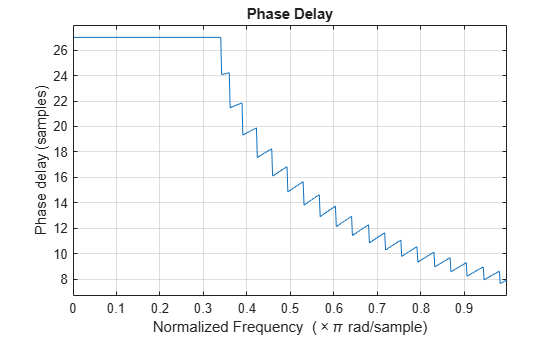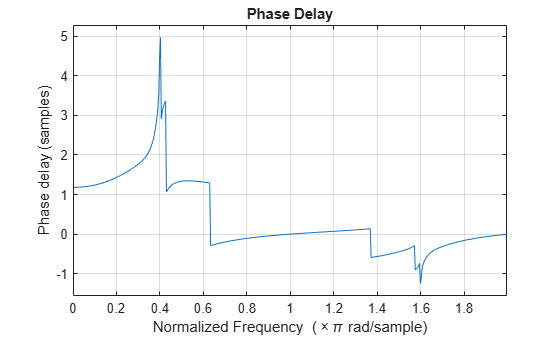Main Content

# phasedelay

Phase delay of digital filter

## Syntax

```[phi,w] = phasedelay(b,a,n) [phi,w] = phasedelay(sos,n) [phi,w] = phasedelay(d,n) [phi,w] = phasedelay(...,n,'whole') phi = phasedelay(...,w) [phi,f] = phasedelay(...,n,fs) [phi,f] = phasedelay(...,n,'whole',fs) phi = phasedelay(...,f,fs) [phi,w,s] = phasedelay(...) [phi,f,s] = phasedelay(...) phasedelay(...) ```

## Description

`[phi,w] = phasedelay(b,a,n)` returns the `n`-point phase delay response vector, `phi`, and the `n`-point frequency vector in radians/sample, `w`, of the filter defined by numerator coefficients, `b`, and denominator coefficients, `a`. The phase delay response is evaluated at `n` equally spaced points around the upper half of the unit circle. If `n` is omitted, it defaults to 512. For best results, set `n` to a value greater than the filter order.

`[phi,w] = phasedelay(sos,n)` returns the `n`-point phase delay response for the second-order sections matrix, `sos`. `sos` is a K-by-6 matrix, where the number of sections, K, must be greater than or equal to 2. If the number of sections is less than 2, `phasedelay` considers the input to be a numerator vector, `b`. Each row of `sos` corresponds to the coefficients of a second order (biquad) filter. The ith row of the `sos` matrix corresponds to ```[bi(1) bi(2) bi(3) ai(1) ai(2) ai(3)]```.

`[phi,w] = phasedelay(d,n)` returns the `n`-point phase delay response of the digital filter, `d`. Use `designfilt` to generate `d` based on frequency-response specifications.

`[phi,w] = phasedelay(...,n,'whole')` uses `n` equally spaced points around the whole unit circle.

`phi = phasedelay(...,w)` returns the phase delay response at frequencies specified, in radians/sample, in vector `w`. The frequencies are normally between 0 and π. `w` must contain at least two elements.

`[phi,f] = phasedelay(...,n,fs)` and ```[phi,f] = phasedelay(...,n,'whole',fs)``` return the phase delay vector `f` (in Hz), using the sampling frequency `fs` (in Hz). `f` must contain at least two elements.

`phi = phasedelay(...,f,fs)` returns the phase delay response at the frequencies specified in vector `f` (in Hz), using the sampling frequency `fs` (in Hz).

`[phi,w,s] = phasedelay(...)` and `[phi,f,s] = phasedelay(...)` return plotting information, where `s` is a structure with fields you can change to display different frequency response plots.

`phasedelay(...)` with no output arguments plots the phase delay response versus frequency.

Note

If the input to `phasedelay` is single precision, the phase delay response is calculated using single-precision arithmetic. The output, `phi`, is single precision.

## Examples

collapse all

Use constrained least squares to design a lowpass FIR filter of order 54 and normalized cutoff frequency 0.3. Specify the passband ripple and stopband attenuation as 0.02 and 0.08, respectively, expressed in linear units. Compute and plot the phase delay response of the filter.

```Ap = 0.02; As = 0.008; b = fircls1(54,0.3,Ap,As); phasedelay(b)```Repeat the example using `designfilt`. Keep in mind that this function expresses the ripples in decibels.

```Apd = 40*log10((1+Ap)/(1-Ap)); Asd = -20*log10(As); d = designfilt('lowpassfir','FilterOrder',54,'CutoffFrequency',0.3, ... 'PassbandRipple',Apd,'StopbandAttenuation',Asd); phasedelay(d)```Design an elliptic filter of order 10 and normalized passband frequency 0.4. Specify a passband ripple of 0.5 dB and a stopband attenuation of 20 dB. Display the phase delay response of the filter over the complete unit circle.

```[b,a] = ellip(10,0.5,20,0.4); phasedelay(b,a,512,'whole')```Repeat the example using `designfilt`.

```d = designfilt('lowpassiir','DesignMethod','ellip','FilterOrder',10, ... 'PassbandFrequency',0.4, ... 'PassbandRipple',0.5,'StopbandAttenuation',20); phasedelay(d,512,'whole')```## See Also

Introduced before R2006a

## Support

#### Deep Learning for Signal Processing with MATLAB

Download white paper